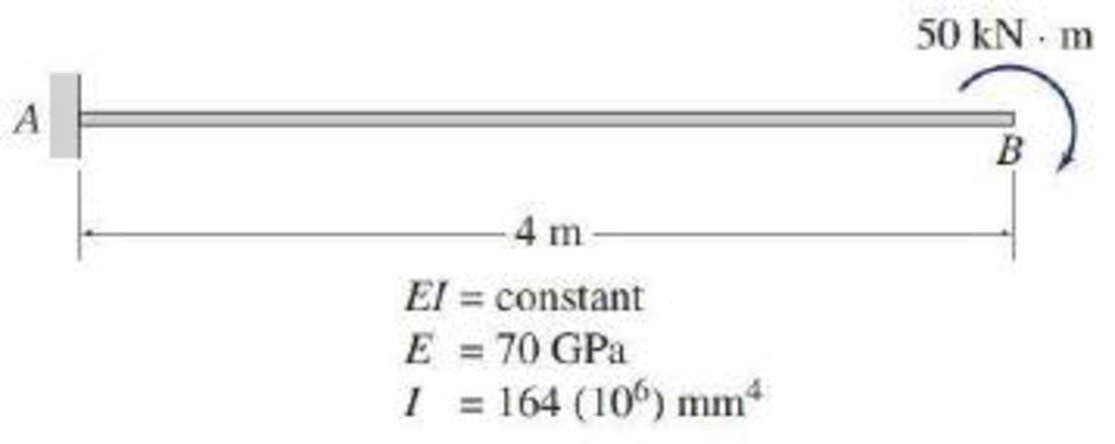# 7.20 and 7.21 Use the virtual work method to determine the slope and deflection at point B of the beam shown. FIG. P7.21, P7.23, P7.58

#### Solutions

Chapter
Section
Chapter 7, Problem 21P
Textbook Problem
227 views

## 7.20 and 7.21 Use the virtual work method to determine the slope and deflection at point B of the beam shown.FIG. P7.21, P7.23, P7.58

To determine

Find the slope and deflection at point B of the beam using virtual work method.

### Explanation of Solution

Given information:

The beam is given in the Figure.

The value of E is 70 GPa and I is 164(106)mm4.

Calculation:

Consider the real system.

Sketch the real system of the beam as shown in Figure 1.

Refer Figure 1.

Consider the section at x distance from the end B.

Calculate the moment M as follows:

M=50

Consider the virtual system.

Remove all the real loads and apply a unit couple at the point on the beam where the slope is desired.

Sketch the virtual system of the beam with unit couple at point B as shown in Figure 2.

Let an equation expressing the variation of bending moment due to virtual couple be Mv1.

Refer Figure 2.

Mv1=1

Find the slope at B using the virtual work expression:

1(θB)=0LMv1MEIdx (1)

Here, L is the length of the beam, E is the Young’s modulus, and I is the moment of inertia.

Substitute 1 for Mv1, 4 for L, and 50 for M in Equation (1).

θB=1EI04(1)(50)dx=1EI04(50)dx=1EI[50x]04=200kNm2EI

Substitute 70 GPa for E and 164(106)mm4 for I.

Therefore, the slope at point B of the beam is 0

### Still sussing out bartleby?

Check out a sample textbook solution.

See a sample solution

#### The Solution to Your Study Problems

Bartleby provides explanations to thousands of textbook problems written by our experts, many with advanced degrees!

Get Started

Find more solutions based on key concepts
When learning to play some sports, such as tennis, golf, or baseball, often you are told to follow through with...

Engineering Fundamentals: An Introduction to Engineering (MindTap Course List)

Differentiate among user names, passwords, passphrases, and pass codes.

Enhanced Discovering Computers 2017 (Shelly Cashman Series) (MindTap Course List)

What should be done to a magnetic chuck when it becomes unevenly worn?

Precision Machining Technology (MindTap Course List)

Using only one equilibrium equation, compute the force in rope AD of Prob. 5.33.

International Edition---engineering Mechanics: Statics, 4th Edition

If your motherboard supports ECC DDR3 memory, can you substitute non-ECC DDR3 memory?

A+ Guide to Hardware (Standalone Book) (MindTap Course List)

What changes can be made to successfully make a weld in a poorly fitted joint?

Welding: Principles and Applications (MindTap Course List)